GRE Subject Test: Math : Evaluating Limits

Example Questions

Example Question #71 : Gre Subject Test: Math

Evaluate: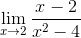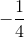Undefined/No LimitExplanation:

Step 1: See if we can plug ininto the equation..

We can't because the denominator becomes..

Step 2: Factor the denominator: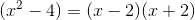(By the Difference of Perfect Squares Formula)

Step 3: Re-write the function: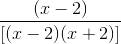Step 4: Divide by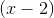on both the numerator and denominator because it's common:

We are left with: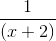Step 5: Plug in: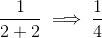The limit of this function as x approaches 2 is.

All GRE Subject Test: Math Resources